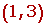Learn from home

The teachers## Increasing

If f is differentiable at a:## Decreasing

If f is differentiable at a:### Calculation of the Intervals of Increase and Decrease

Study the intervals of increase and decrease of:

f(x) = x³ − 3x + 2

To determine the intervals of increase and decrease, perform the following steps:

1. Differentiate the function.

f'(x) = 3x² −3

2. Obtain the roots of the first derivative: f'(x) = 0.

3x² −3 = 0 x = -1 x = 1

3. Form open intervals with the zeros (roots) of the first derivative and the points of discontinuity (if any).4. Take a value from every interval and find the sign they have in the first derivative.

If f'(x) > 0 is increasing.

If f'(x) < 0 is decreasing.

On the interval (−∞, −1), take x = −2, for example.

f'(2) = 3(2)² −3 > 0

On the interval (−1, 1), take x = 0, for example.

f'(0) = 3(0)² −3 < 0

On the interval (1, ∞), take x = 2, for example.

f'(2) = 3(2)² −3 > 05. Write the intervals of increase and decrease:

Increasing: (−∞, −1) (1, ∞)

Decreasing: (−1,1)

## Example of the Intervals of Increase and DecreaseDid you like the article?(4 votes, average: 5.00 out of 5)Loading...

Emma

I am passionate about travelling and currently live and work in Paris. I like to spend my time reading, gardening, running, learning languages and exploring new places.

Did you like
this resource?

Bravo!